### An Overview of Convolutional Neural Networks

Convolutional Neural Networks are a specific type of neural network systems that are particularly suited for object recognition/ computer vision problems. In such tasks, the dataset is represented as a 2-Dimensional grid of pixels. See Figure 1.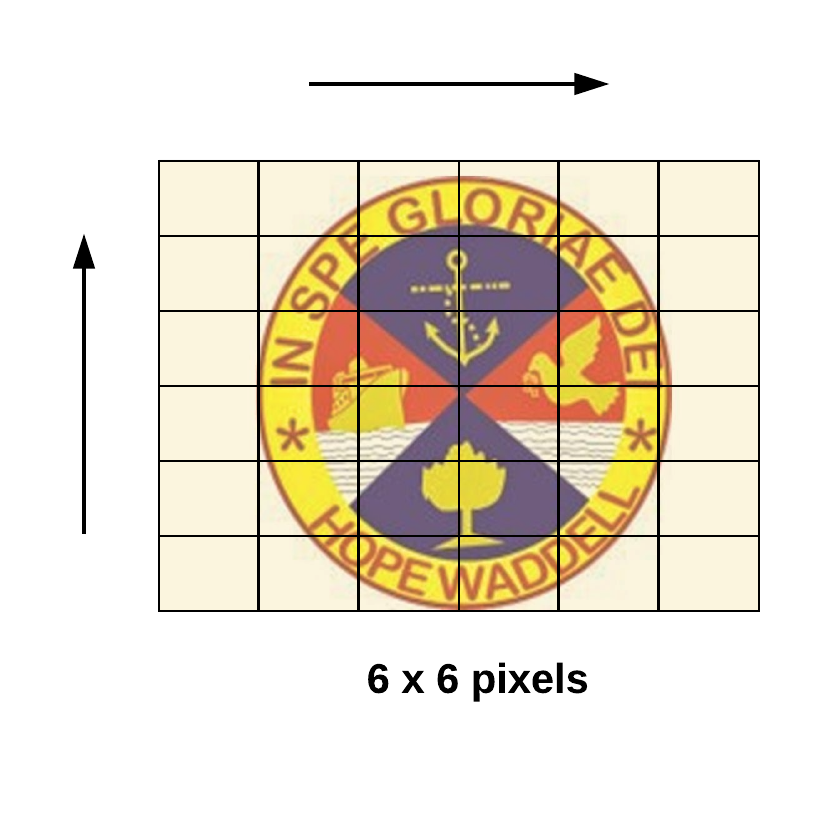Figure 1: 2-D representation of an image.

An image is depicted in the computer as a matrix of pixel intensity values ranging from 0 - 255. A grayscale (or black and white) image consists of a single channel with 0 representing the black areas and 255 the white regions with the values in-between for various shades of grey. For example, the image below is a 10 x 10 grayscale image with its matrix representation.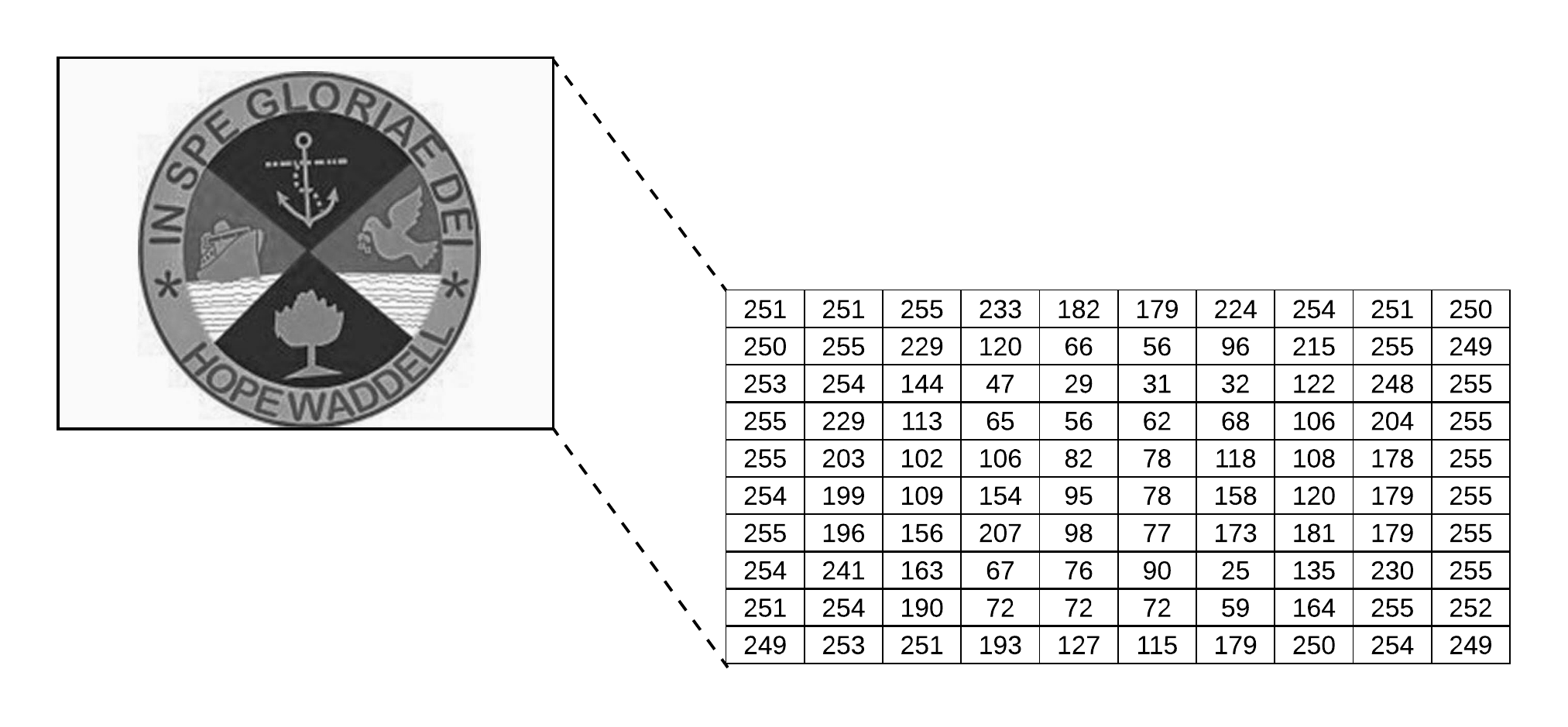Figure 2: Grayscale image with matrix representation.

On the other hand, a colored image consists of three channels, Red, Green, and Blue, with each channel also containing pixel intensity values from 0 to 255. A colored image has a matrix shape of [height x width x channel]. In the example below, we have an image of shape [10 x 10 x 3] indicating a 10 x 10 matrix with three channels.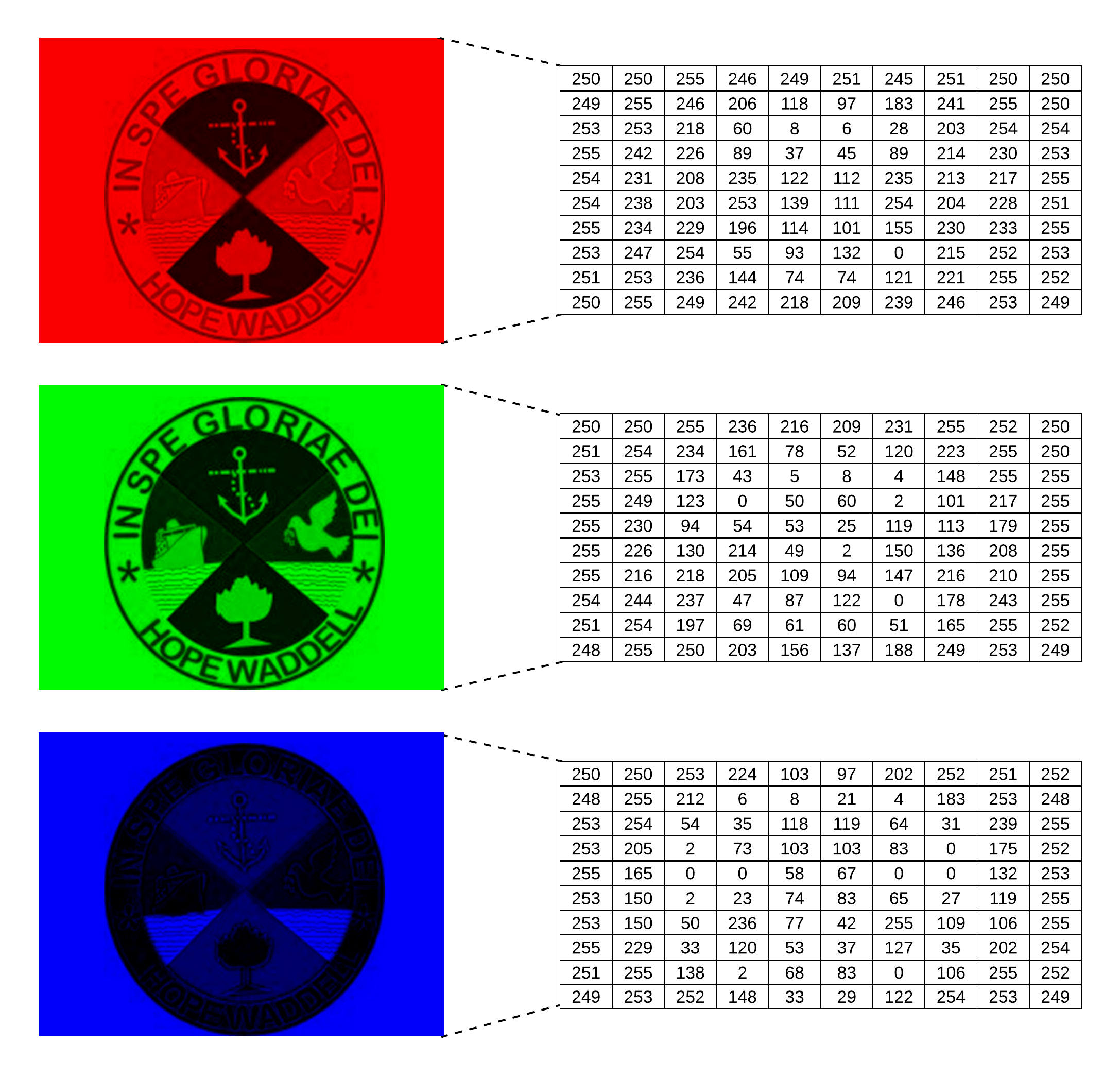Figure 3: Colored image with matrix representation.

### Local Receptive Fields of the Visual Cortex

The core concept of Convolutional Neural Networks is built on understanding the local receptive fields founds in the neurons of the visual cortex - the part of the brain responsible for processing visual information.

A local receptive field is an area on the neuron that excites or activates that neuron to fire information to other neurons. When viewing an image, the neurons in the visual cortex react to a small or limited area of the overall image due to the presence of a small local receptive field. Hence, the neurons in the visual cortex do not all sense the entire image at the same time, but they are activated by viewing a local area of the image via its local receptive field.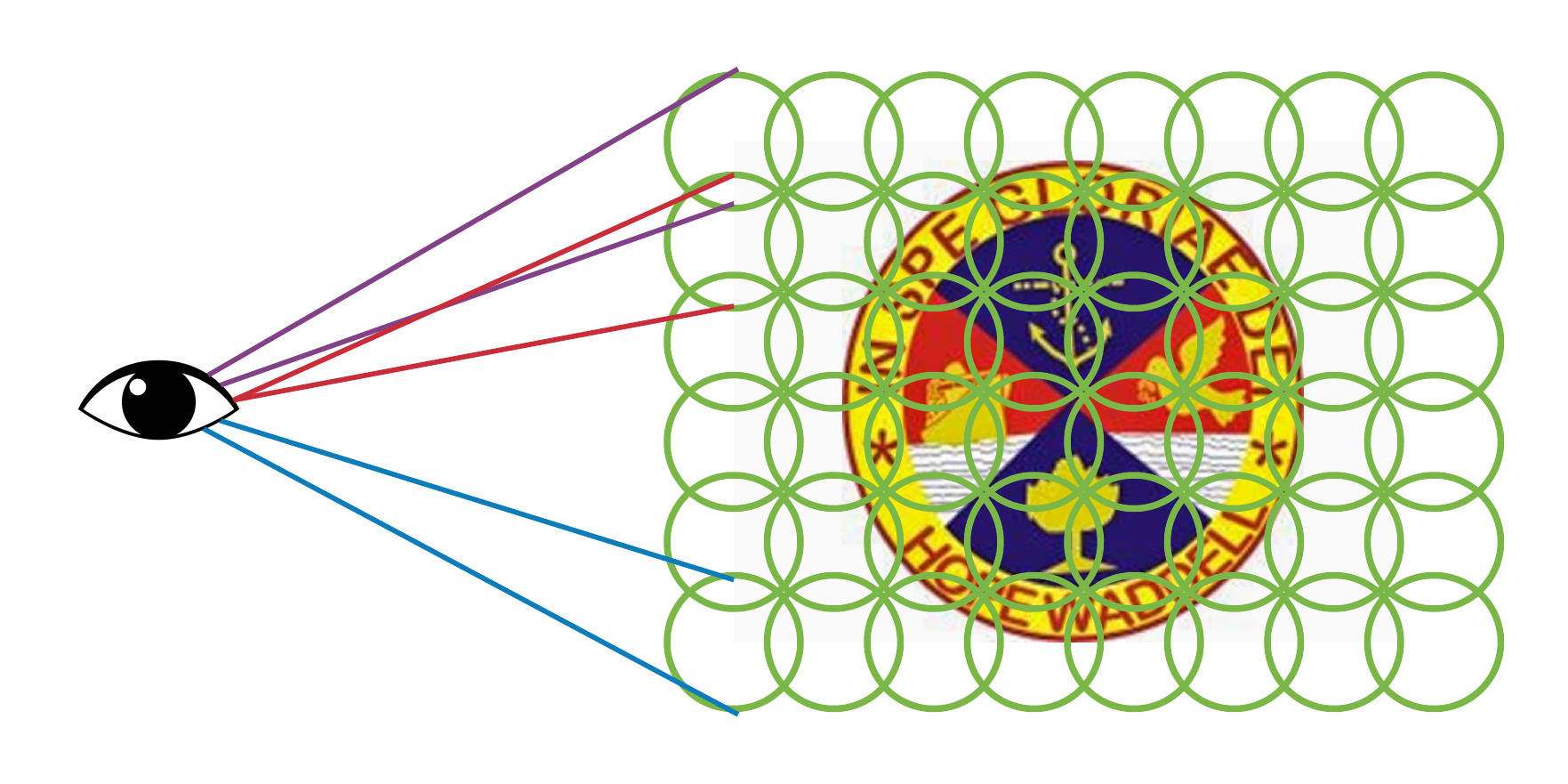Figure 4: Local Receptive Field.

From the image above, the local receptive fields overlap to give a collective perspective on the entire image. Each neuron in the visual cortex reacts to a different type of visual information (e.g., lines with different orientations). Moreso, other neurons have large receptive fields that react to more complex visual patterns such as edges, regions, etc. From here we get the idea that neurons with larger receptive field receive information from those with lower receptive fields as they progressively learn the visual information of the image.

Suppose we have a 28 x 28 pixel set of image data, a feedforward neural network or multi-layer perceptron will need 784 input weights plus a bias. By flattening an image in this way, we lose the spatial relationship of the pixels in the image.

CNN’s on the other hand, can learn complex image features by preserving the spatial relationship between the image pixels. It does so by stacking convolutional layers whereby the neurons in the higher layers with a larger receptive field receive information from neurons in the lower layers having a smaller receptive field. So CNN learns a hierarchy of increasingly complex features from the input data as it flows through the network.

In CNN, the neurons (or filters) in the convolutional layer are not all connected to the pixels in the input image as we have in the dense multi-layer perceptron. Hence, CNN is also called a sparse neural network. A distinct advantage of CNN over MLP is the reduced number of weights needed for training the network. Convolutional Neural Networks are composed of three fundamental types of layers, namely:

• Convolutional Layer:: The Convolution layer is made up of filters and feature maps. A filter is passed over the input image pixels to capture a specific set of features in a process called convolution. The output of a filter is called a feature map.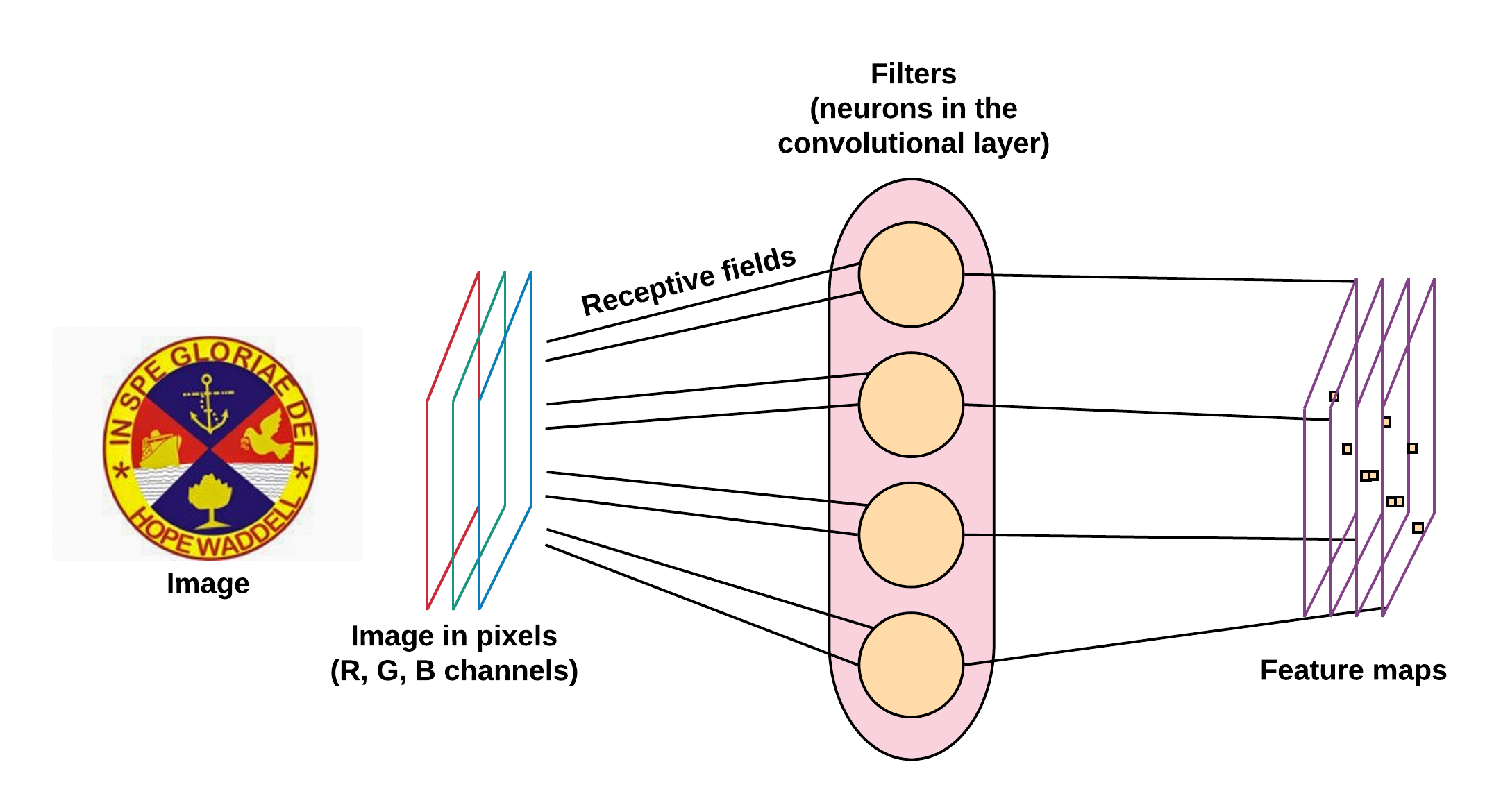Figure 5: The Convolution Process.
• Pooling Layer: Pooling layers typically follow one or more convolutional layers. The goal of the Pooling layer is to reduce or downsample the feature map of the convolutional layer. The Pooling layer summarizes the image features learned in the previous network layers. By doing so, it also helps prevent the network from overfitting. Moreso, the reduction in the input size also bodes well for processing and memory costs when training the network.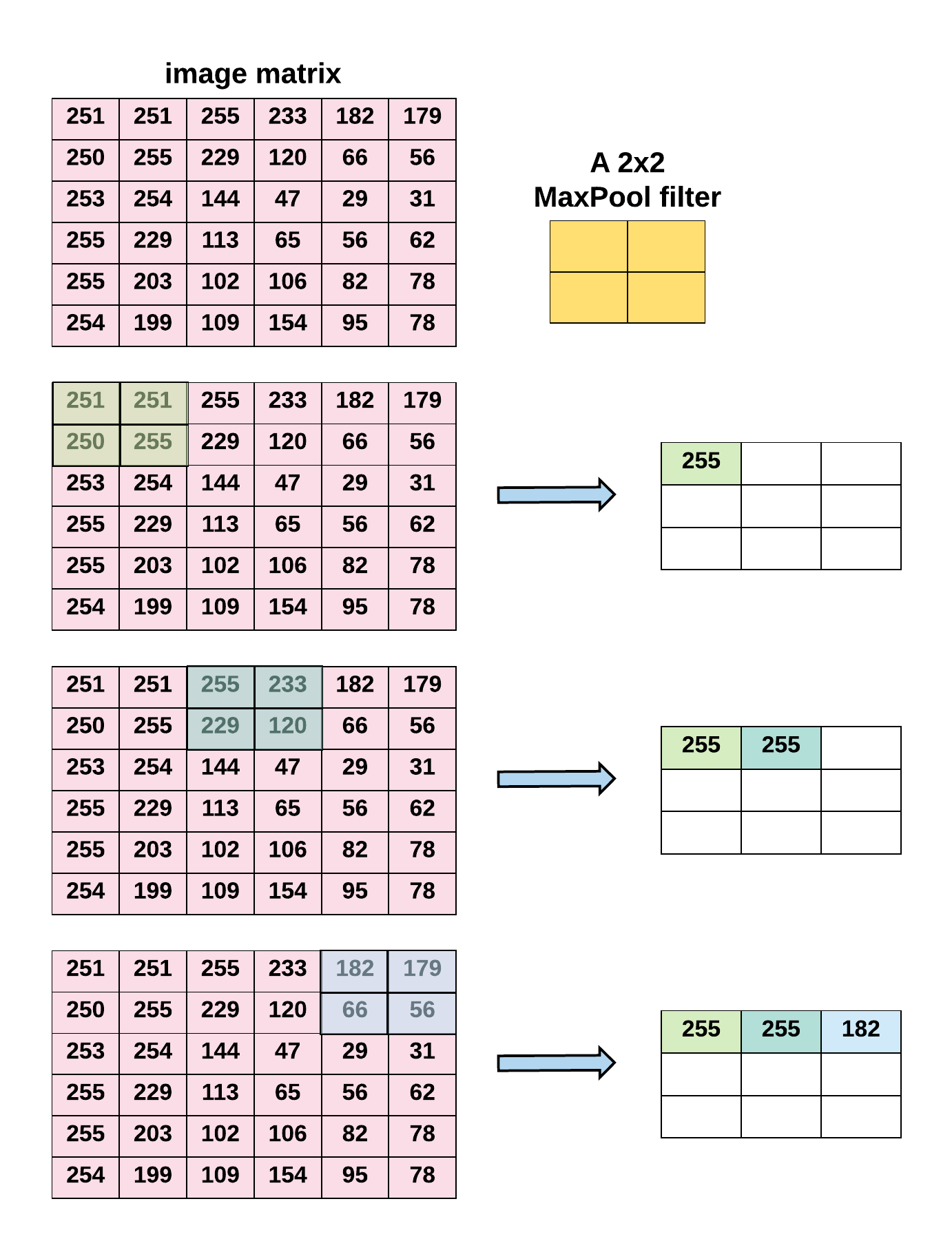Figure 6: Example of Pooling with MaxPooling.
• Fully Connected Layer: The Fully Connected Network (FCN) layer is our regular feed-forward neural network or multi-layer perceptron. These layers typically have a non-linear activation function. In any case, the FCN is the final layer of the Convolutional Neural Network. In this case, a softmax activation is used to output the probabilities that an input belongs to a particular class. Before passing an input into the FCN, the image matrix will have to be flattened. For example, a 28 x 28 x 3 image matrix will become a 2,352 input weights plus a bias of 1 into the fully connected network.Figure 7: CNN Architecture.